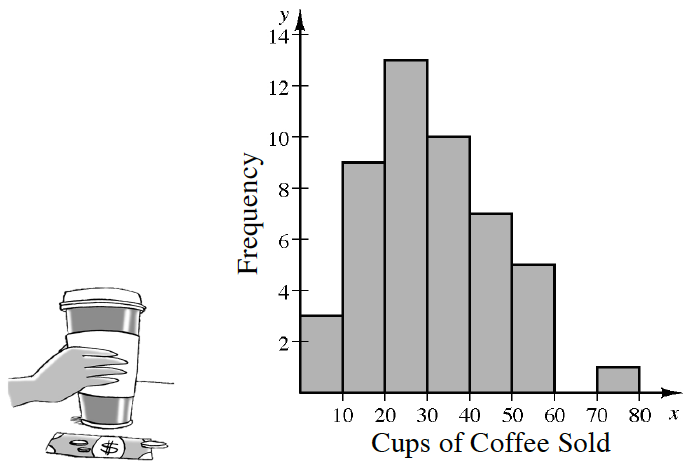### Home > CCA2 > Chapter 9 > Lesson 9.1.1 > Problem9-8

9-8.

Katelyn owns a $24$-hour coffee shop in front of a downtown office building. She recorded the number of cups of coffee sold each hour during a $48$-hour time period.

Katelyn sorted the data and made the following histogram:1.  Using the sorted data from the table and the histogram, find the five number summary (minimum, first quartile, median, third quartile, maximum) without using your calculator.

For quartiles:
Look between the $19$ and the $20$.
Look between the $29$s.
Look between the $40$ and the $41$.

Minimum: $1$
Maximum: $76$

1. Describe the distribution of coffee sales per hour.

What would be the typical number of cups sold in an hour?
Does the distribution have skew? Is there an outlier?
What is the IQR? What would it represent?

 Cups of Coffee Sold $1$ $22$ $37$ $5$ $22$ $38$ $7$ $23$ $38$ $12$ $24$ $40$ $12$ $25$ $41$ $12$ $26$ $41$ $12$ $26$ $42$ $12$ $29$ $47$ $14$ $29$ $47$ $16$ $30$ $49$ $17$ $30$ $51$ $19$ $31$ $52$ $20$ $32$ $55$ $21$ $33$ $55$ $21$ $35$ $59$ $22$ $36$ $76$ checksum $1444$